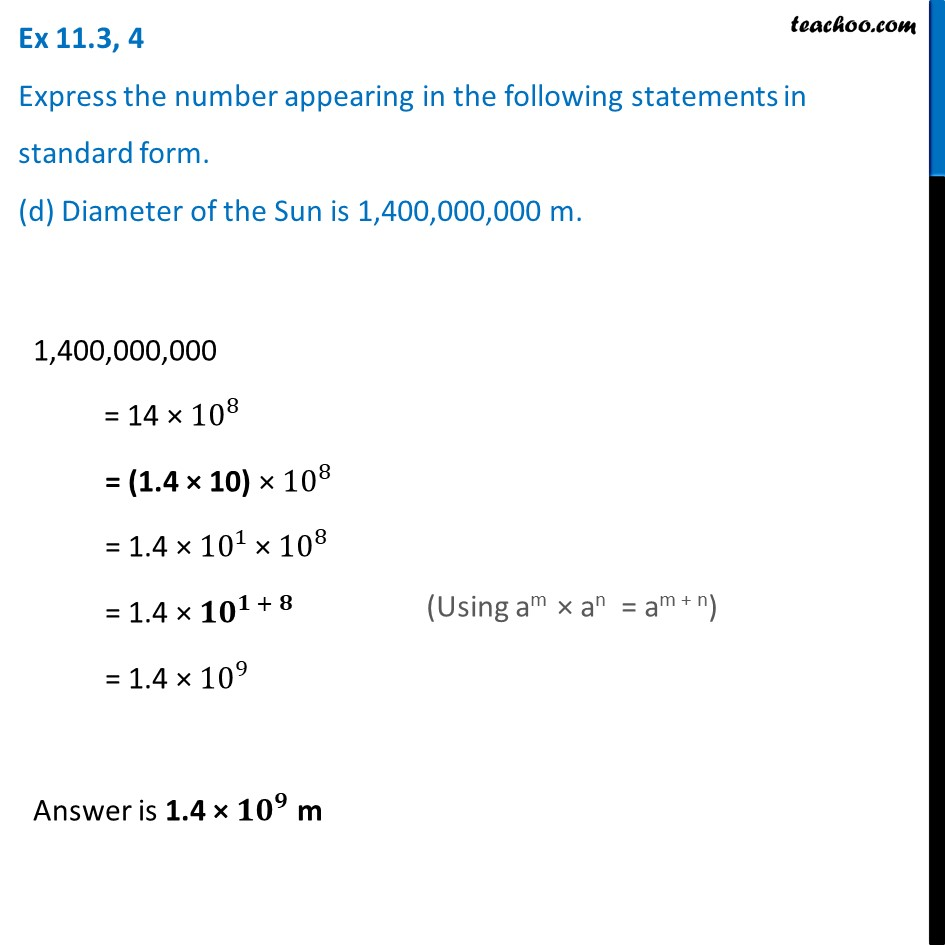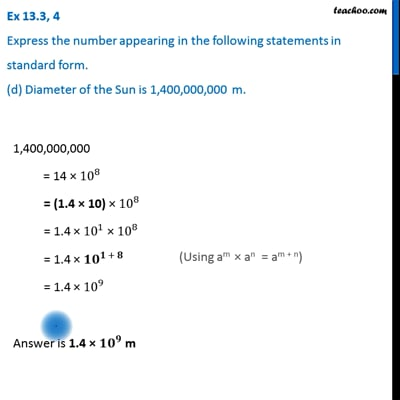Ex 11.3

Chapter 11 Class 7 Exponents and Powers
Serial order wiseThis video is only available for Teachoo black users

Learn in your speed, with individual attention - Teachoo Maths 1-on-1 Class

### Transcript

Ex 11.3, 4 Express the number appearing in the following statements in standard form. (d) Diameter of the Sun is 1,400,000,000 m. 1,400,000,000 = 14 × 〖10〗^8 = (1.4 × 10) × 〖10〗^8 = 1.4 × 〖10〗^1 × 〖10〗^8 = 1.4 × 〖10〗^(1 + 8) = 1.4 × 〖10〗^9 Answer is 1.4 × 〖𝟏𝟎〗^𝟗 m (Using am × an = am + n)